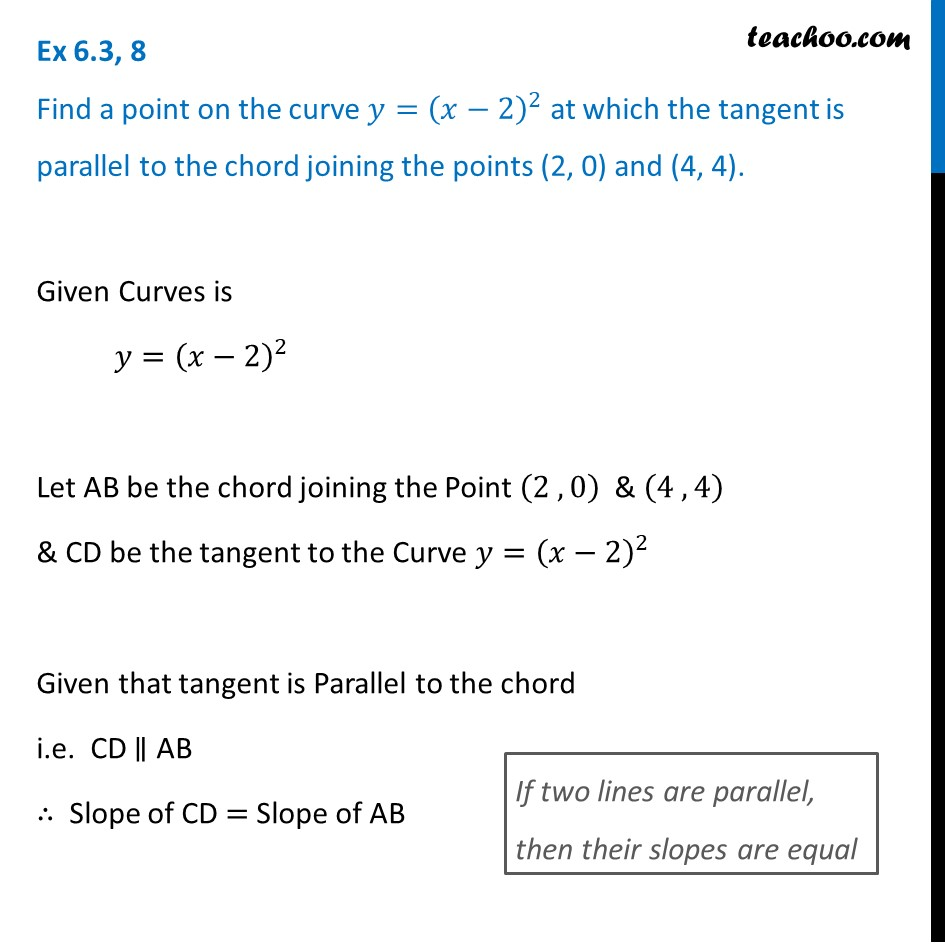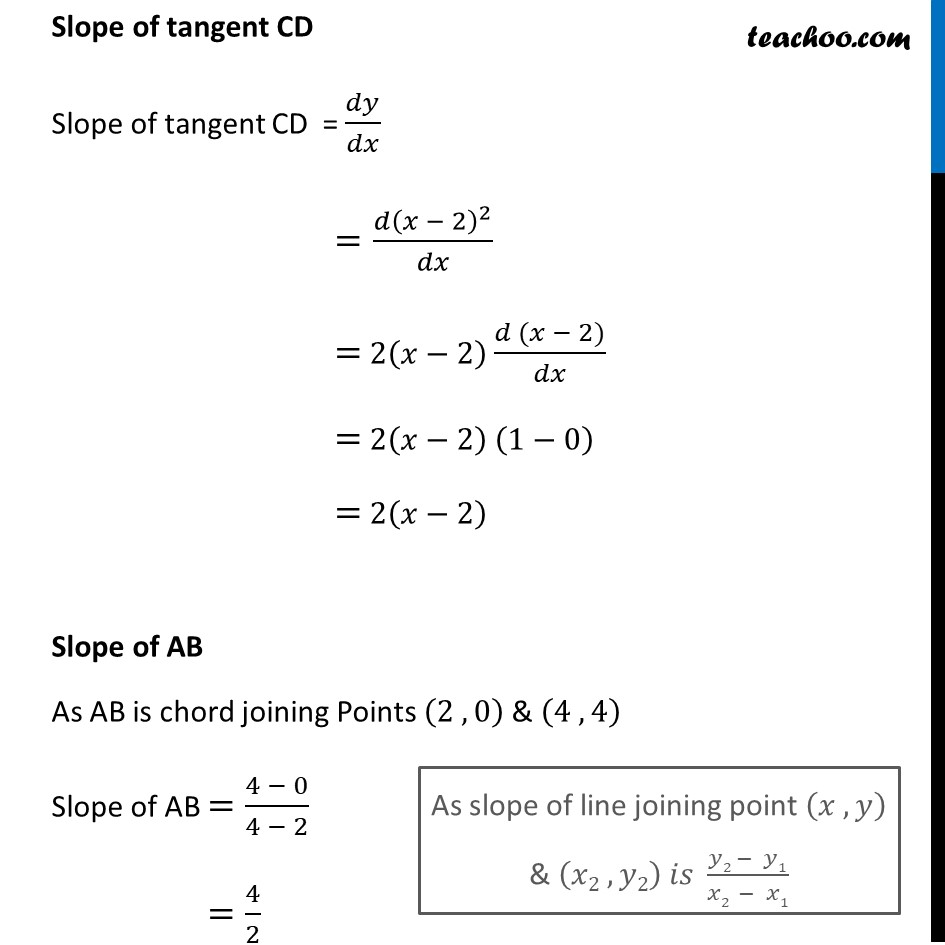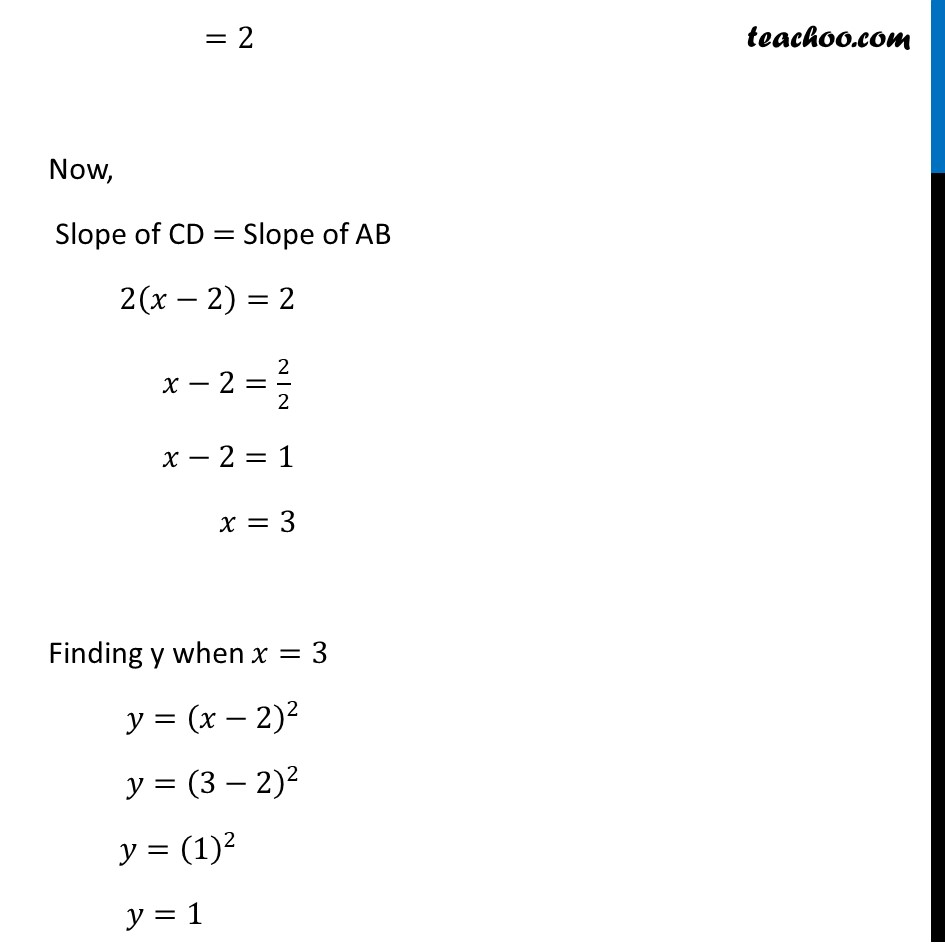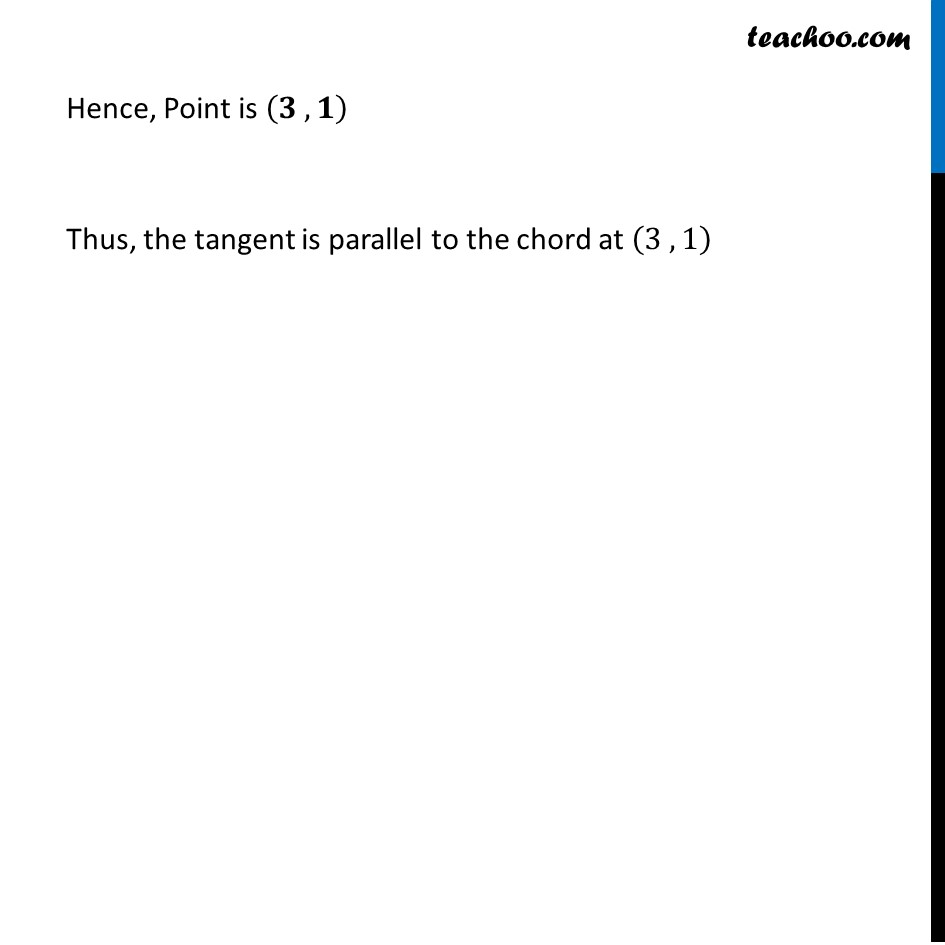Tangents and Normals (using Differentiation)

Chapter 6 Class 12 Application of Derivatives
Serial order wiseLearn in your speed, with individual attention - Teachoo Maths 1-on-1 Class

### Transcript

Question 8 Find a point on the curve 𝑦=(𝑥−2)^2 at which the tangent is parallel to the chord joining the points (2, 0) and (4, 4).Given Curves is 𝑦=(𝑥−2)^2 Let AB be the chord joining the Point (2 , 0) & (4 ,4) & CD be the tangent to the Curve 𝑦=(𝑥−2)^2 Given that tangent is Parallel to the chord i.e. CD ∥ AB ∴ Slope of CD = Slope of AB If two lines are parallel, then their slopes are equal Slope of tangent CD Slope of tangent CD = 𝑑𝑦/𝑑𝑥 =(𝑑(𝑥 − 2)^2)/𝑑𝑥 = 2(𝑥−2) (𝑑 (𝑥 − 2))/𝑑𝑥 = 2(𝑥−2) (1−0) = 2(𝑥−2) Slope of AB As AB is chord joining Points (2 , 0) & (4 , 4) Slope of AB =(4 − 0)/(4 − 2) =4/2 As slope of line joining point (𝑥 , 𝑦) & (𝑥2 , 𝑦2) 𝑖𝑠 (𝑦2 − 𝑦1)/(𝑥2 − 𝑥1) =2 Now, Slope of CD = Slope of AB 2(𝑥−2)=2 𝑥−2=2/2 𝑥−2=1 𝑥=3 Finding y when 𝑥=3 𝑦=(𝑥−2)^2 𝑦=(3−2)^2 𝑦=(1)^2 𝑦=1 Hence, Point is (𝟑 , 𝟏) Thus, the tangent is parallel to the chord at (3 ,1)